×

Search anything:

# Josephus Problem

#### Algorithms List of Mathematical AlgorithmsGet this book -> Problems on Array: For Interviews and Competitive Programming

In this article, we have explored and solved Josephus Problem in depth which is a Standard Problem in Computer Science. We have presented two solutions to Josephus Problem.

1. Introduction to Josephus Problem
2. Solving the Josephus Problem
3. Implementation of Recursive Solution
4. An Alternative Solution

## Introduction to Josephus Problem

The Josephus Problem is a theoretical problem in computer science and mathematics, where people standing in a circle get executed one-by-one until one person remains. A person at a specified position starts counting, and this counting proceeds in one direction, until it reaches a pre-fixed number, after which the person standing next in the circle gets executed. The counting restarts from the next person, and this process continues until all but one person remains.

The problem is usually stated in terms of the number of persons standing in the circle (n), and a number k, which denotes that the kth person will be executed on counting.

Toy Case

We shall first consider a toy case, where n = 7, and k = 2.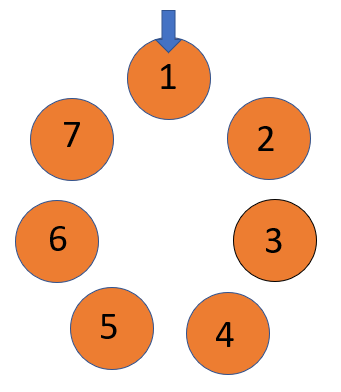First, since 1 starts the counting and the kth person gets executed, 2 gets executed.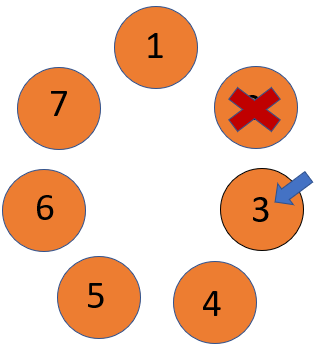Now, since 3 starts counting, 4 gets executed, and in a similar manner 6 also gets executed once 5 starts counting.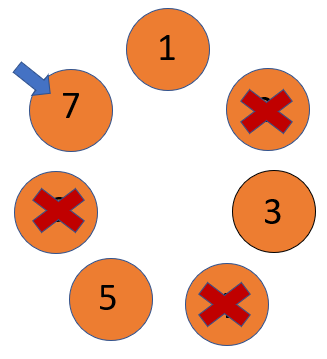Now, since it is 7 who starts counting, 1 gets executed. Now the count goes to 3, and 5 gets executed.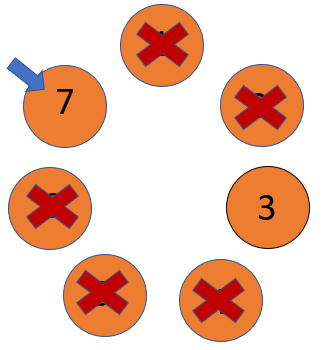The count again goes to 7, and hence 3 gets executed.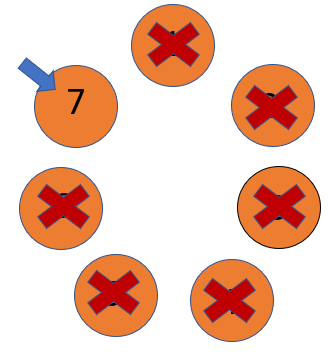Since 7 is the only person remaining, he wins.

## Solving the Josephus Problem

Let us denoted the Josephus problem with n people and k counts as J(n,k). We first observe the following:

• The problem eliminates one person at a time until 1 person remains.
• Let the position of the person who starts counting be i. Then the person executed would be (i+ (k-1))(mod n) Here, we add k-1 since the counting starts from and includes the ith person. Also, we obtain the remainder modulo n since after the last person counts, it would once again be the first person in the circle who continues the count. In this case, the position of the person who starts counting next would be (i + (k-1))(mod n) + 1.
• After the first person gets executed, J(n,k) gets reduced to J(n-1, k), but the start position in this case becomes (1 + k - 1)(mod n) + 1.
• When the problem is J(1,k), then only one person remains, and hence J(1,k) = 1.

Hence, we can observe that J(n,k) can be broken down into smaller subproblems, and can therefore be solved recursively. We define the recursive relation as follows:

J(n,k) = (J(n-1,k) + k-1)(mod n) + 1, with J(1,k) = 1

Since this recursive call runs exactly n times, and since the number of calls does not depend on k, the time complexity of this solution is given by O(n). The space complexity is also O(n), since each recursion takes O(1) space, and the total number of recursions is n.

Toy Case Revisited

We now try to solve J(7,2) using the recursive formula dervied earlier. We shall work backwards from J(1,2).
Now,
J(1,2) = 1
J(2,2) = [J(1,2) + 1](mod 2) + 1 = 1
J(3,2) = [J(2,2) + 1](mod 3) + 1 = 3
J(4,2) = [J(3,2) + 1](mod 4) + 1 = 1
J(5,2) = [J(4,2) + 1](mod 5) + 1 = 3
J(6,2) = [J(5,2) + 1](mod 6) + 1 = 5
J(7,2) = [J(6,2) + 1](mod 7) + 1 = 7

Hence, we have obtained the same solution as earlier.

## Implementation of Recursive Solution

We shall now implement this using C++.

``````#include<iostream>
using namespace std;

int JP(int n, int k)
{
if(n==1) return 1;
else return (JP(n-1,k) + 1) % n + 1;
}

int main()
{
int n = 10, k =4;
cout<<JP(n,k);
}
``````

Here, we observe that J(10,4) = 5, which can be verified manually.

## An Alternative Solution

Although the recursive solution is efficient, there is always a chance of stack overflow, since it may need a lot of memory to hold the intermediate solutions. This problem can be resolved with an iterative implementation of the same solution. In this method, we shall work backwards from the case where there are only two people. We calculate the position of the winner in each case, and thus determine the winner for the given scenario. Note that the logic here is the same as that of the recursive solution.

Since this iterative loop shall run n-1 times, it's time complexity is given by O(n), the same as the recursive solution. However, the space complexity is O(1), since the loop does not need any extra memory.

Implementation of Iterative Solution

``````#include<iostream>
using namespace std;

int JP2(int n, int k)
{
int pos = 1;
for(int i = 2; i<=n; i++)
{
pos = (pos + k - 1) % i + 1;
}
return pos;
}

int main()
{
int n = 7, k = 2;
cout<<JP2(n,k);
}
``````

With this article at OpenGenus, you must have the complete idea of Josephus Problem.#### Aravind Mohandas

Aravind Mohandas is a Master's student at Indian Institute of Technology, Ropar with B.Sc in Computer Science from Christ University, Bangalore. He is an intern at OpenGenus.